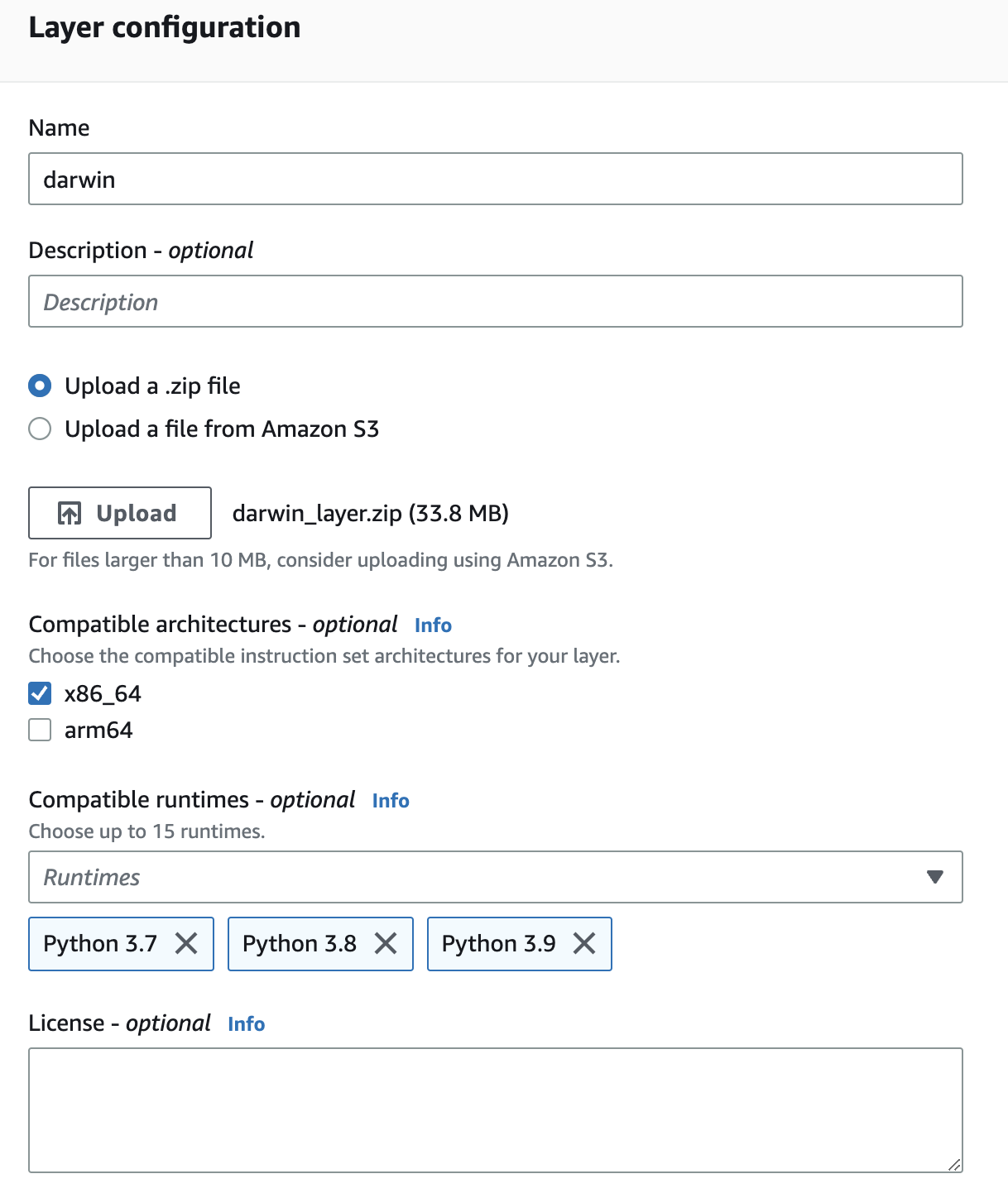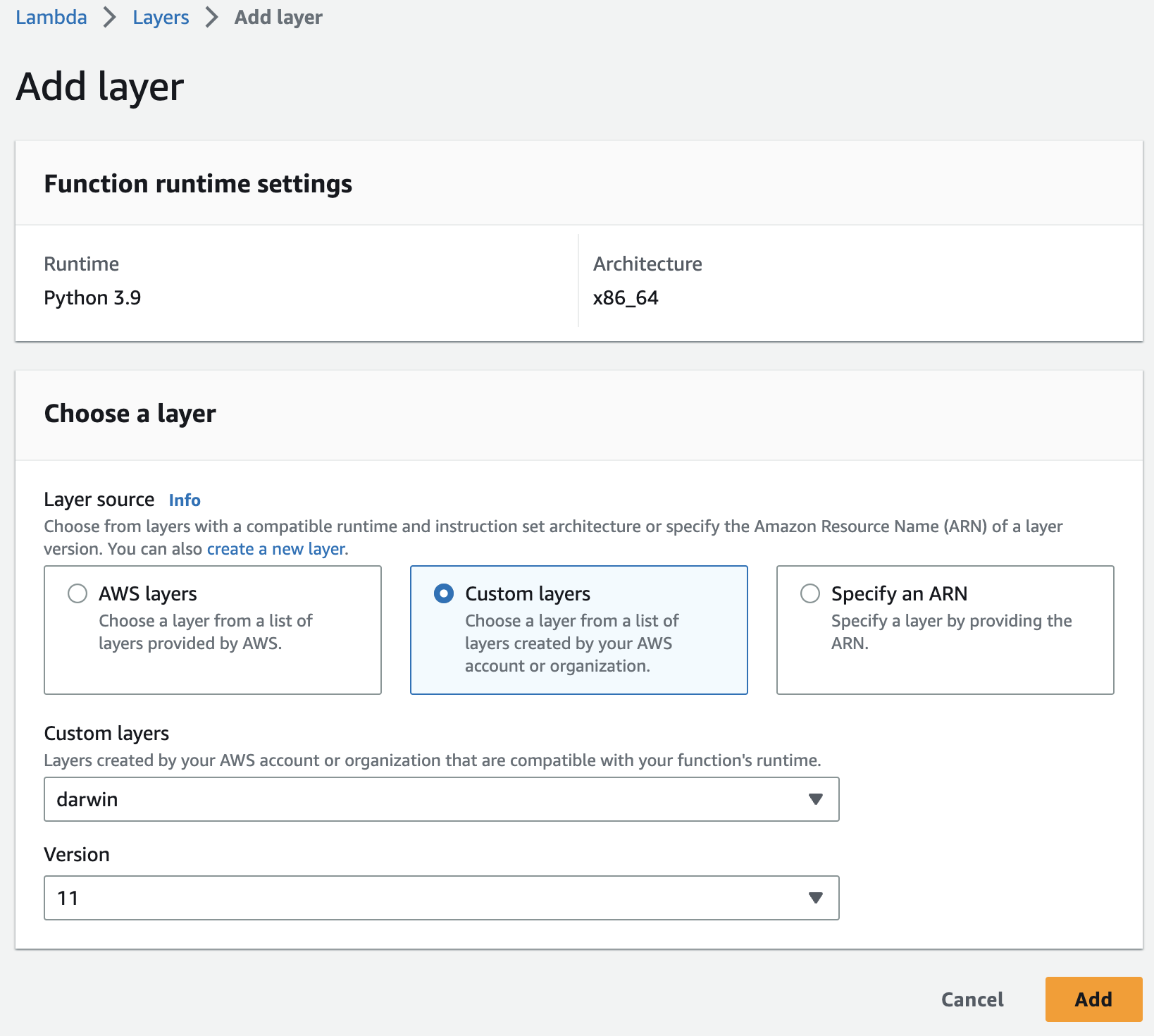# Using darwin-py with AWS Lambda

A guide to using V7's darwin-py library in AWS Lambda

Due to some dependencies in the darwin-py library being composed of compiled code, it is necessary to take some extra steps when uploading it on AWS Lambda if your OS is not Amazon Linux.

Follow the steps in this guide to get darwin-py working as a Lambda layer.

## 1: Installing required packages

First, execute each group of shell commands below to install the required packages and create zip archives of each one:

numpy:

``````pip install --target=./python --platform manylinux2010_x86_64 --only-binary=:all: numpy
zip -r numpy_layer.zip python
rm -rf ./python/
``````

upolygon:

``````pip install --target=./python --platform manylinux2010_x86_64 --only-binary=:all: upolygon
zip -r upolygon_layer.zip python
rm -rf ./python/
``````

pillow:

``````pip install --target=./python --platform manylinux2010_x86_64 --only-binary=:all: pillow
zip -r pillow_layer.zip python
rm -rf ./python/
``````

darwin-py: Note that we remove `mpire`, a multiprocessing package that does not work in AWS Lambda.

``````pip install --target=./python --platform manylinux2014_x86_64 --only-binary=:all: darwin-py==0.8.21
rm -rf ./python/mpire
zip -r darwin_layer.zip python
rm -rf ./python/
``````

📘

Supported darwin-py versions

darwin-py as a Lambda layer is currently supported for versions 0.8.21 and 0.8.22. You can change the version you would like to use as a layer by adjusting the shell command above.

In AWS Lambda, navigate to Layers and for each zip file created above, follow the below steps:

• 1: Select Create Layer, then fill in "Name" as desired
• 2: Check `x86_64` under "Compatible architectures"
• 3: Select the desired compatible runtime(s). Note that Python versions 3.7, 3.8, & 3.9 are supported
• 4: Select Upload then select the relevant zip file
• 5: Select CreateNavigate to "Functions" within AWS Lambda and select the function to add the layers to. For each layer just created, follow the below steps:

• 1: Scroll down to the "Layers" section then select Add a layer
• 2: Select Custom layers then select the layer to be added. Note that numpy, pillow, upolygon and darwin-py all need to be added as layers
• 3: Select the available version under "Version", then select Add

For example, when adding the darwin-py layer to a Lambda running a Python 3.9 runtime, your settings should appear as:To test that the layers are working, you can deploy the below code to your Lambda and test.

``````import darwin
import json
import numpy as np

def lambda_handler(event, context):
# Create a 2x2 random matrix
random_matrix = np.random.rand(2, 2)

# Compute the matrix inverse
matrix_inverse = np.linalg.inv(random_matrix)

response = {
'statusCode': 200,
'body': json.dumps({
'random_matrix': random_matrix.tolist(),
'matrix_inverse': matrix_inverse.tolist()
})
}

return response
``````

If successful, you will receive a 200 statusCode response and a random numpy matrix. For example:

``````Response
{
"statusCode": 200,
"body": "{\"random_matrix\": [[0.9439886223271938, 0.8518401925330076], [0.2962384992804775, 0.8611787653255121]], \"matrix_inverse\": [[1.5361867620302543, -1.519528441507594], [-0.528435766558139, 1.6839045312248049]]}"
}
``````